# ormap and filter CS 5010 Program Design Paradigms

• Slides: 20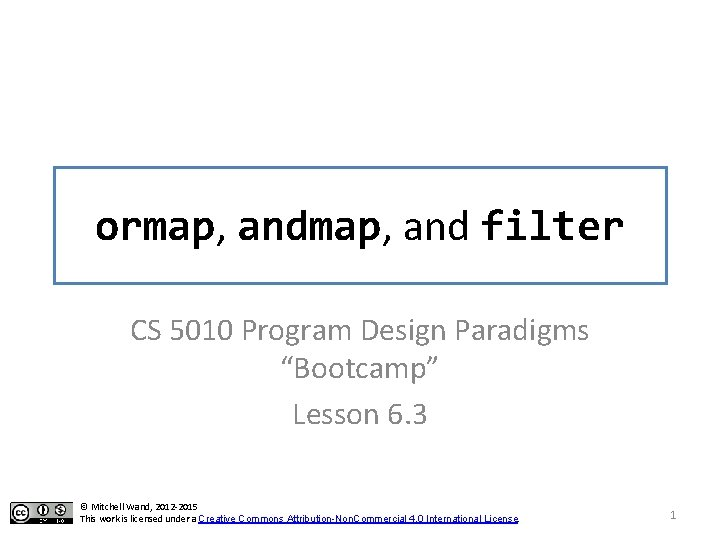Introduction • In this lesson, we will see more common patterns of function definitions that differ only by what functions they call. 2Learning Objectives • At the end of this lesson you should be able to: – recognize the ormap, and filter patterns – state the contracts for ormap, and filter, and use them appropriately. – combine these functions using higher-order function combination 3Let's look at find-dog again ; ; find-dog : String. List -> Boolean ; ; RETURNS: true if "dog" is in the given list. ; ; STRATEGY: Use template for String. List on lst (define (find-dog lst) (cond [(empty? lst) false] [else (or (string=? (first lst) "dog") (find-dog (rest lst)))])) (check-equal? (find-dog (list "cat" "dog" "weasel")) true) (check-equal? (find-dog (list "cat" "elephant" "weasel")) false) 4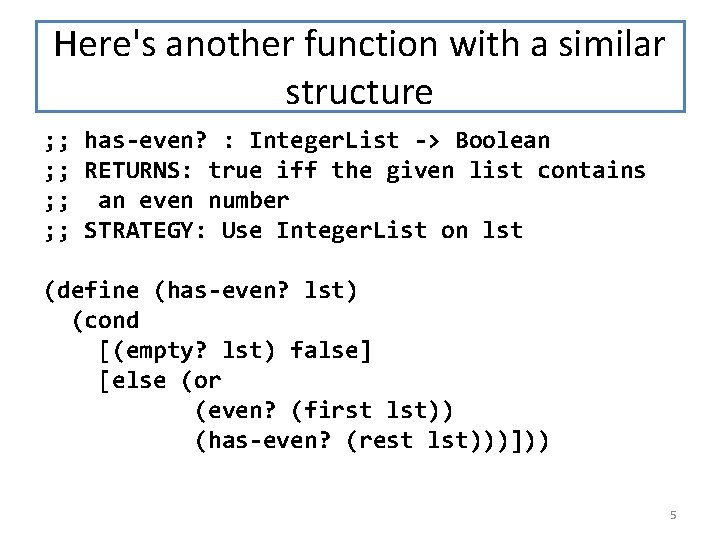Here's another function with a similar structure ; ; has-even? : Integer. List -> Boolean ; ; RETURNS: true iff the given list contains ; ; an even number ; ; STRATEGY: Use Integer. List on lst (define (has-even? lst) (cond [(empty? lst) false] [else (or (even? (first lst)) (has-even? (rest lst)))])) 5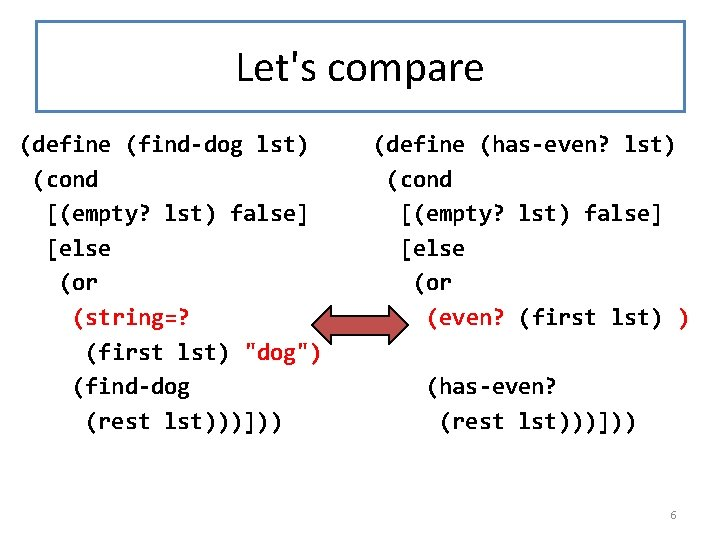Let's compare (define (find-dog lst) (cond [(empty? lst) false] [else (or (string=? (first lst) "dog") (find-dog (rest lst)))])) (define (has-even? lst) (cond [(empty? lst) false] [else (or (even? (first lst) ) (has-even? (rest lst)))])) 6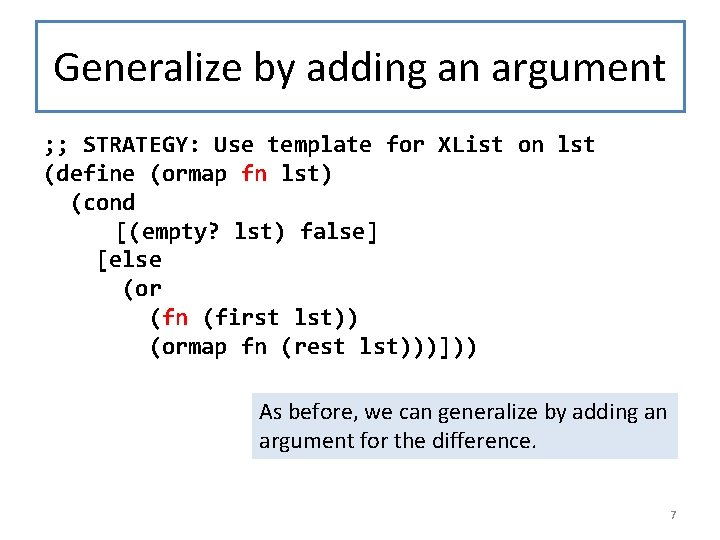Generalize by adding an argument ; ; STRATEGY: Use template for XList on lst (define (ormap fn lst) (cond [(empty? lst) false] [else (or (fn (first lst)) (ormap fn (rest lst)))])) As before, we can generalize by adding an argument for the difference. 7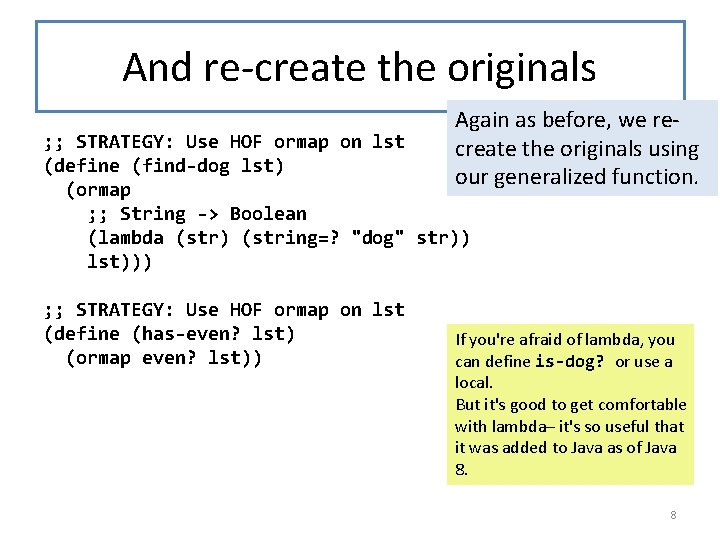And re-create the originals Again as before, we recreate the originals using our generalized function. ; ; STRATEGY: Use HOF ormap on lst (define (find-dog lst) (ormap ; ; String -> Boolean (lambda (str) (string=? "dog" str)) lst))) ; ; STRATEGY: Use HOF ormap on lst (define (has-even? lst) (ormap even? lst)) If you're afraid of lambda, you can define is-dog? or use a local. But it's good to get comfortable with lambda– it's so useful that it was added to Java as of Java 8. 8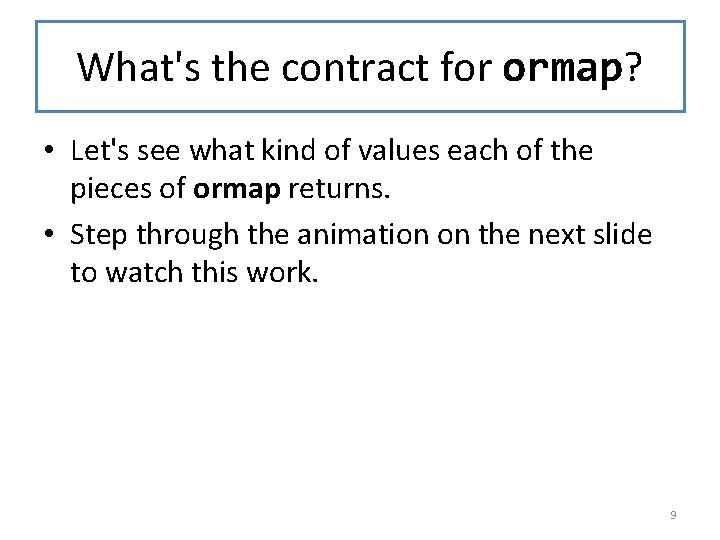What's the contract for ormap? • Let's see what kind of values each of the pieces of ormap returns. • Step through the animation on the next slide to watch this work. 9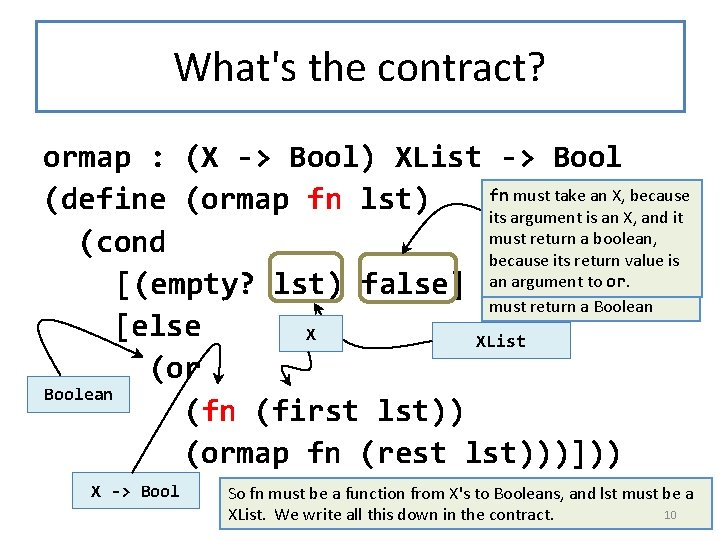What's the contract? ormap : (X -> Bool) XList -> Bool fn must take an X, because (define (ormap fn lst) its argument Boolean is an X, and it must return a boolean, (cond because its return value is Both branches of the cond an argument to or. booleans, so ormap [(empty? lst) false] return must return a Boolean [else X XList (or Boolean (first lst)) (ormap fn (rest lst)))])) X -> Bool So fn must be a function from X's to Booleans, and lst must be a 10 XList. We write all this down in the contract.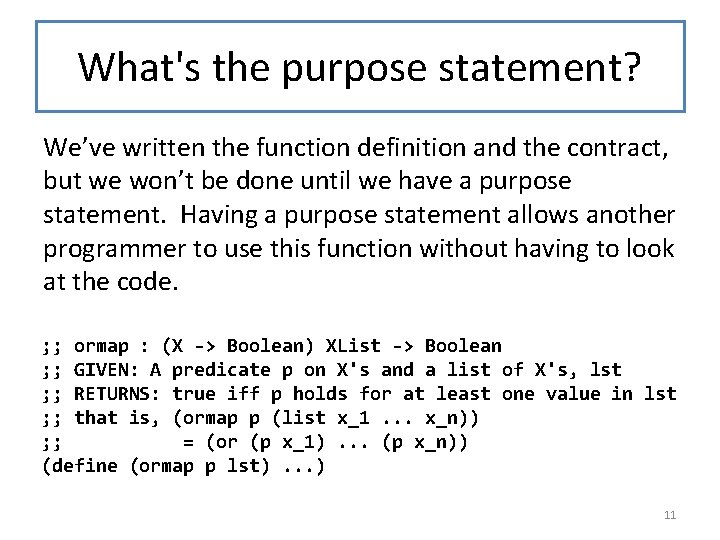What's the purpose statement? We’ve written the function definition and the contract, but we won’t be done until we have a purpose statement. Having a purpose statement allows another programmer to use this function without having to look at the code. ; ; ormap : (X -> Boolean) XList -> Boolean ; ; GIVEN: A predicate p on X's and a list of X's, lst ; ; RETURNS: true iff p holds for at least one value in lst ; ; that is, (ormap p (list x_1. . . x_n)) ; ; = (or (p x_1). . . (p x_n)) (define (ormap p lst). . . ) 11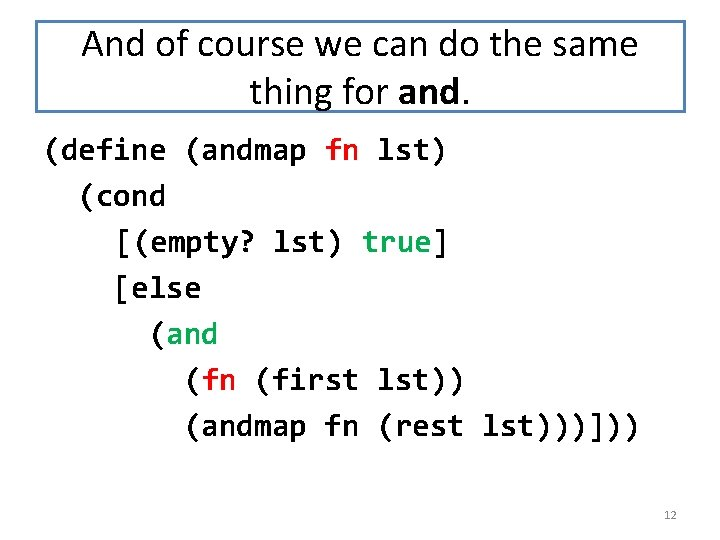And of course we can do the same thing for and. (define (andmap fn lst) (cond [(empty? lst) true] [else (and (fn (first lst)) (andmap fn (rest lst)))])) 12Contract and Purpose Statement ; ; ; ; andmap : (X -> Bool) XList -> Bool GIVEN: A predicate p on X's and a list of X's, lst RETURNS: true iff p holds for every value in lst that is, (andmap p (list x_1. . . x_n)) = (and (p x_1). . . (p x_n)) The contract and purpose statement look very much like the ones for ormap. 13Another common pattern • Another common list-manipulation problem is to take a list and return a list of those values in the list that pass a certain test. • For example, here's a function that returns only the even values in a list of integers. 14only-evens ; ; : Integer. List -> Integer. List ; ; returns the list of all the even values ; ; in the list ; ; STRATEGY: Use template for Integer. List on lst (define (only-evens lst) (cond [(empty? lst) empty] [else (if (even? (first lst)) (cons (first lst) (only-evens (rest lst)))])) 15Generalize: filter ; ; filter : (X -> Boolean) XList ; ; -> XList ; ; RETURNS: the list of all the elements ; ; in the list that satisfy the test ; ; STRATEGY: Use template for XList on lst The obvious thing to (define (filter fn lst) (cond do here is to replace [(empty? lst) empty] even? with an extra [else (if (fn (first lst)) argument. (cons (first lst) (filter fn (rest lst)))])) 16These can be strung together ; ; Integer. List -> Integer. List ; ; RETURNS: the squares of the ; ; evens in the given list ; ; STRATEGY: Use HOF filter on lon, ; ; followed by HOF map (define (squares-of-evens lon) (map sqr One of the nice things (filter even? lon))) about these functions is that they can be combined to create multi-pass functions. 17Go crazy with these! ; ; STRATEGY: Use HOF filter on lon, ; ; followed by HOF map twice (define (squares-of-evens+1 lon) (map add 1 (map sqr (filter even? lon)))) But always make sure that your definitions are CLEAR AND UNDERSTANDABLE! 18Summary • You should now be able to: – recognize the ormap, and filter patterns – state the contracts for ormap, and filter , and use them appropriately. – combine these functions to form more complicated operations on lists. 19Next Steps • Study 06 -2 -1 -map. rkt in the examples folder. • If you have questions about this lesson, ask them on the Discussion Board • Do Guided Practice 6. 3 • Go on to the next lesson 20